Get instant live expert help with Excel or Google Sheets“My Excelchat expert helped me in less than 20 minutes, saving me what would have been 5 hours of work!”

#### Post your problem and you’ll get Expert help in seconds.

Your message must be at least 40 characters
Our professional Expert are available now. Your privacy is guaranteed.

# Discover How to Use the OR Function of Conditional Formatting

Conditional Formatting is an excellent way to visualize the data based on certain criteria. OR function in the Conditional Formatting highlights the data in the table if at least one of the defined conditions is met. This step by step tutorial will assist all levels of Excel users in creating a Conditional Formatting OR formula rule.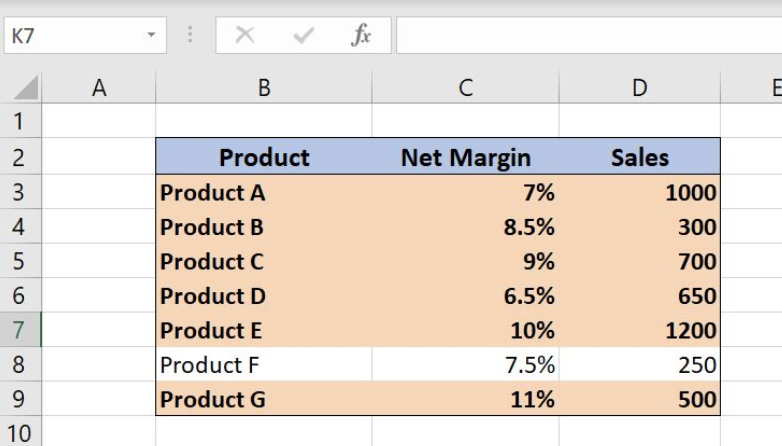Figure 1. Final result

## Syntax of the OR formula

`=OR(logical1,[logical2], …)`

The parameters of the OR function are:

• Logical1, logical2 – the conditions that we want to test

The output of the formula is value TRUE if just one condition is met. If neither of the conditions is met formula result will be FALSE

## Highlight cell values using OR function

To mark the rows in the table based on the certain criteria we can use formula rules in the Conditional Formatting. In our example, we want to emphasize the rows in the table where Net Margin is over 8% or Sales are over 600. For this purpose, we will use OR function since it’s enough that only one condition is met.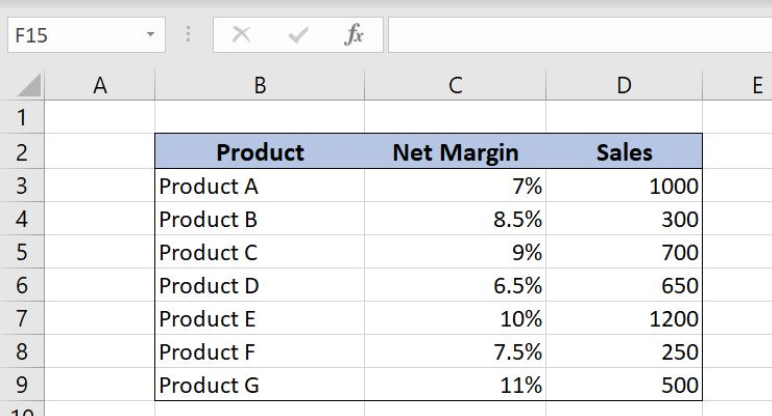Figure 2. Highlight rows where Net Margin is over 8% or Sales is over 600

## Create an OR formula rule in the Conditional Formatting

To create a Conditional Formatting rule based on the formula we should follow the steps below:

• Select the cell, cell range or table in Excel where we what to apply the Conditional Formatting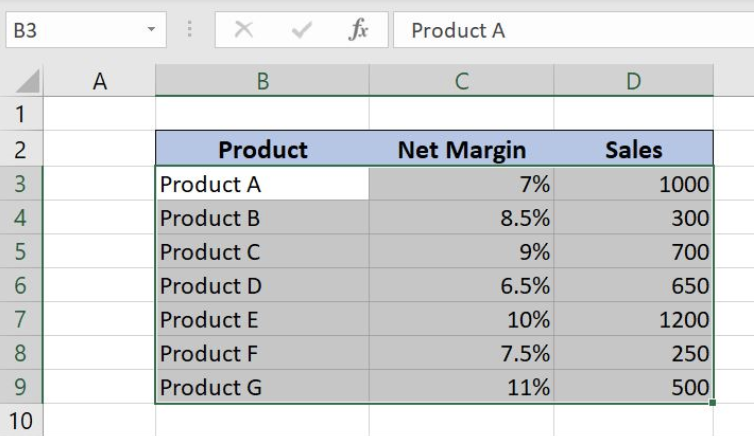Figure 3. Select a cell range for the Conditional Formatting

• Find Conditional Formatting button tab and choose New Rule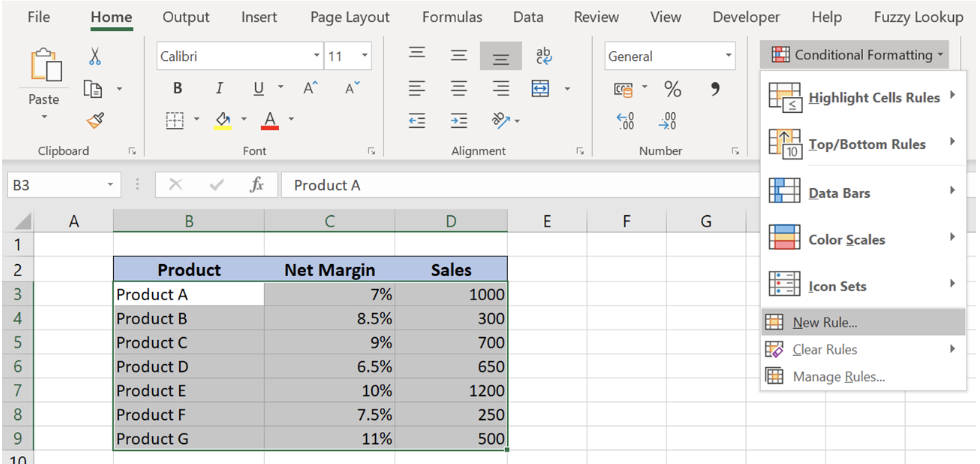Figure 4. Create a new rule in the Conditional Formatting

• Choose Use a formula to determine which cells to format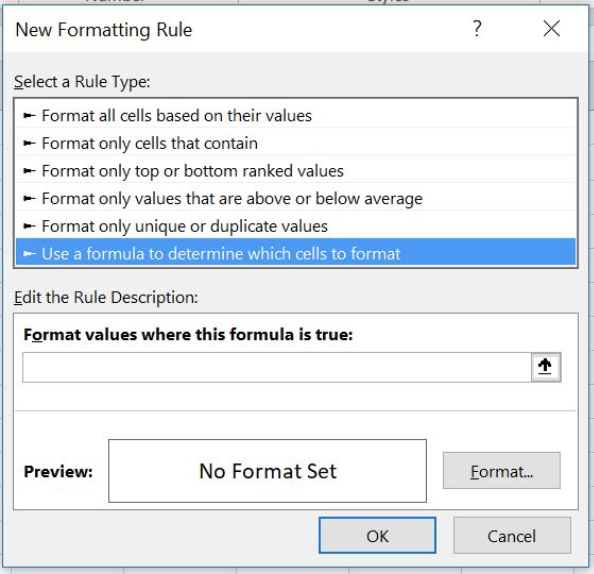Figure 5. Create a formula rule in Conditional Formatting

• Enter formula rule under the section Format values where this formula is true

`=OR(\$C3>8%,\$D3>600)`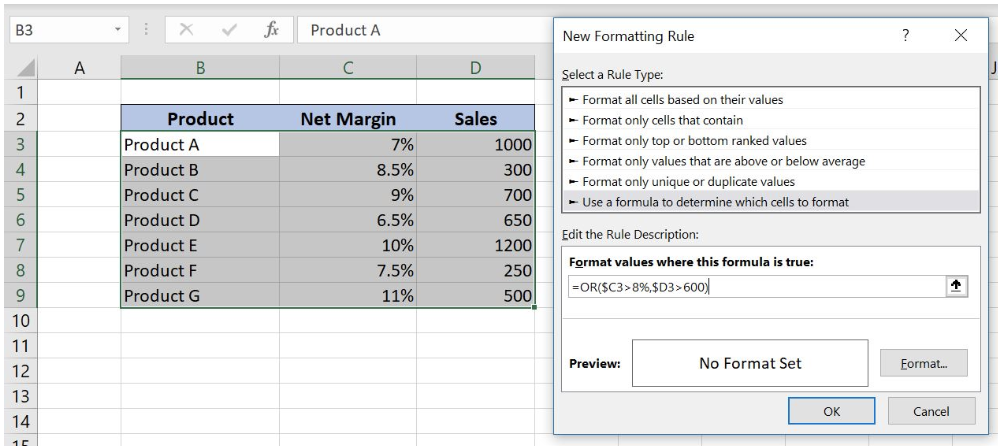Figure 6. OR function in Conditional Formatting

The formula above highlights the rows in the example table where Net Margin is over 8% or Sales is over 600. OR function checks if at least one condition is met and returns the value TRUE. This value triggers the Conditional Formatting rule.

In our OR formula example there are two logical tests:

• Logical1 is \$C3>8% – checks if Net Margin is over 8%
• Logical2 is \$D3>600 – examines if Sales is over 600
• Under the Format tab, we can define the visual appearance of the cells if the OR formula output is TRUE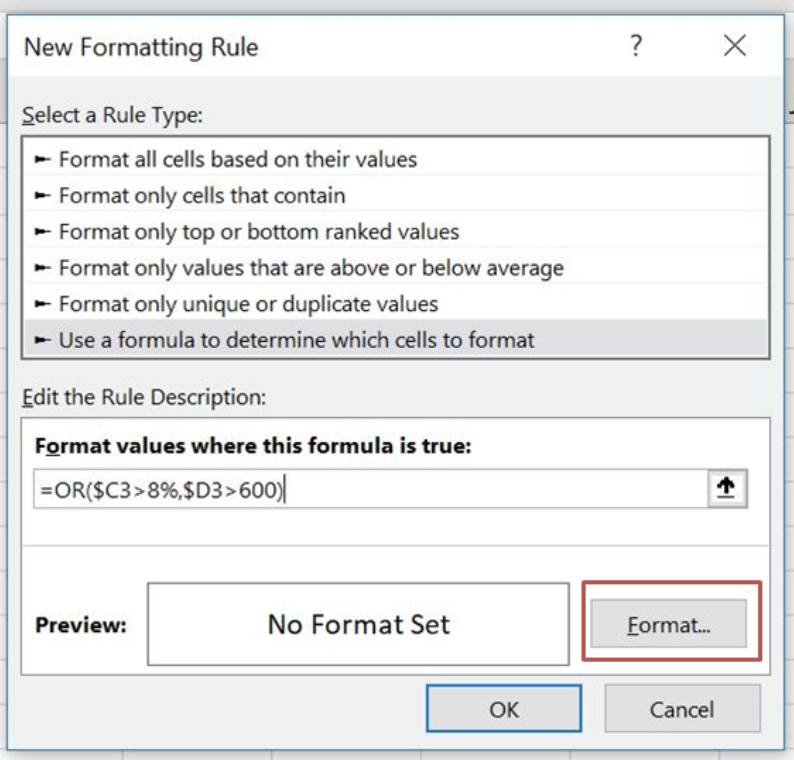Figure 7. Create a custom format in Conditional Formatting

• In Fill tab choose the background color (here you can also choose pattern style and color)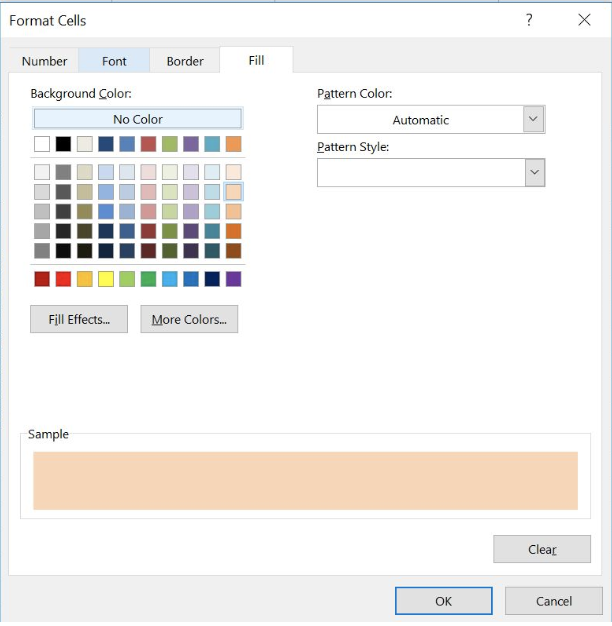Figure 8. Define a Background Color of the cells

• In the Font tab we can define font style and Bold cell text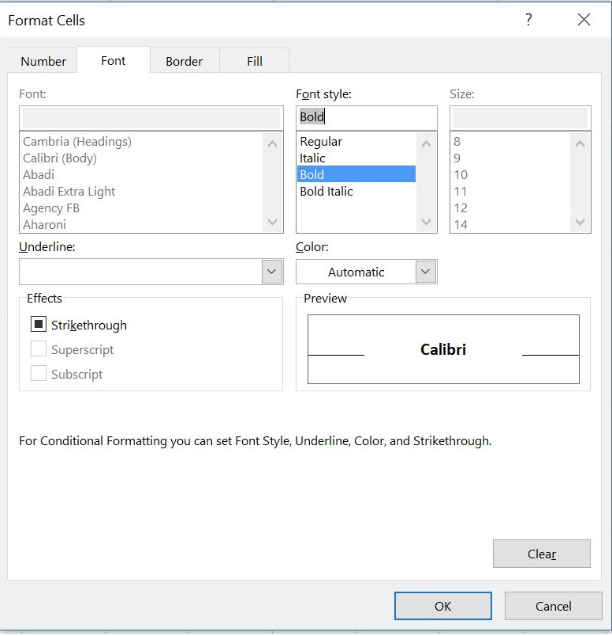Figure 9. Choose a Font style of the cells

• After choosing the format in section Preview we can see how Conditional Formatting cells will look like if the rule is met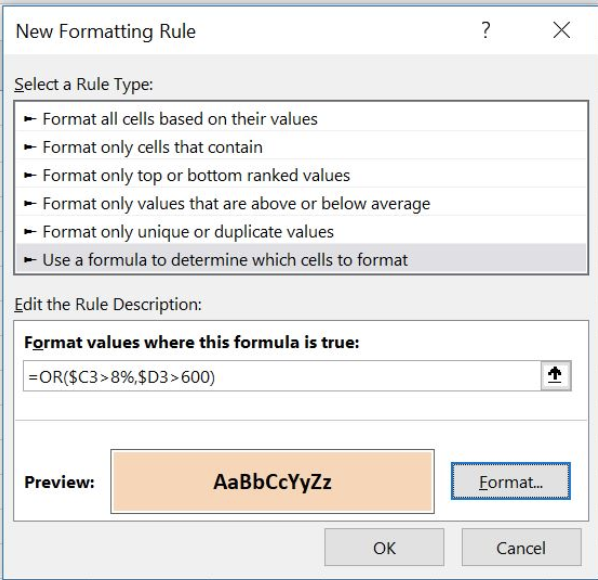Figure 10. Conditional Formatting rule Preview

• Rows in the table are highlighted whenever the Net Margin is over 8% or Sales is over 600. Product F in the table is the only product that meets neither Net Margin nor Sales condition.Figure 11. Conditional Formatting OR formula rule- Net Margin over 8% or Sales over 600

Most of the time, the problem you will need to solve will be more complex than a simple application of a formula or function. If you want to save hours of research and frustration, try our live Excelchat service! Our Excel Experts are available 24/7 to answer any Excel question you may have. We guarantee a connection within 30 seconds and a customized solution within 20 minutes.

### Did this post not answer your question? Get a solution from connecting with the expert.Another blog reader asked this question today on Excelchat:
Solution examplesUse the Vlookup Function to complete the "employee" column of table 2. Use "job Id" from table 2 as your lookup_value(s) and table 1 as your reference.
Solved by C. H. in 16 minsIf a cell in another sheet is populated I need a vlookup done. If the cell is not populated I need the cell to return blank.
Solved by T. D. in 60 minsI am trying to make a chart that turns a week range red if nothing is entered in the range. If something is entered then I would like it to turn green. Please Help
Solved by E. U. in 43 minsI need a check box to show/hide an answer of an if function
Solved by Z. U. in 23 minsI have a list or people who are ranked in numbers from 3 to 6 I need to recognize the contents of each multiple cells and create an equivalent letter. for that value into another column. e.g. Cell E1 = 3 to show in new cell that row (H1) the letter "C" I have multiple rows with different values in column "E" Can this be done?
Solved by I. J. in 30 mins## Subscribe to Excelchat.coAnother blog reader asked this question today on Excelchat: